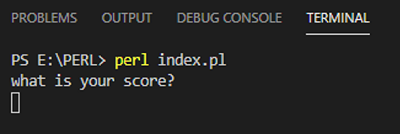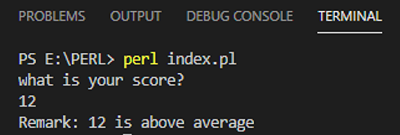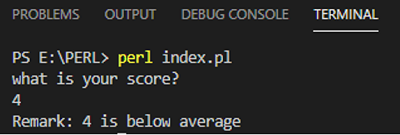# If conditional statement in Perl

In this tutorial, we are going to learn about the If conditional statement in Perl programming language.
Submitted by Godwill Tetah, on December 03, 2020

In programming, there are times you may need to perform a specific task based on conditions. For example, if a student's mark is greater than ten, his remark is above average and if it is below then, it is below average. So to code this kind of program, you will need a conditional statement. Such conditions have two responses, either yes! or no!.

This conditional statement equally exists in other programming languages too and has similar syntax like that of the Perl language.

Today, we are going to see how we can write conditional statements in Perl? Writing conditional statements in Perl is very simple and straight forward to understand.

Syntax:

```if (condition){
#code to run if condition is true
} else {
#code to run if condition is false
}
```

Above is the syntax how the if conditional statement is written in Perl language. Now let's implement the example I gave above.

We will write code that will accept user input (number) using the command <STDIN> and store in a variable which be evaluated later using the condition given.

Our code will evaluate if the number entered is greater than ten or less and remark will be given above average and below average respectively.

After getting user from input, we will remove the "line break" the chomp command. I will suggest you run the code with chomp and without chomp command to better understand the purpose of the chomp command in the code.

Code:

```#Gettings data from a user in Perl

print "what is your score? \n";
\$x = <STDIN>;

chomp(\$x);

if ( \$x > 10 ) {
print "Remark: \$x is above average";
}
else {
print "Remark: \$x is below average";
}
```

Output:

```RUN 1:
15
Remark: 15 is above average

RUN 2:
5
Remark: 5 is below average
```